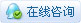2110浏览

# 配对求和问题

 【启智导学】计算：1+2+3+4+5+6+7+8+9+10……n的和请你编程解决这个问题。 【分析提示】我们可以问题具体化，例如累加求和到10，同时发现将最小的数字1和最大的数字10配对，和为11；将2和9配对，和也是11……这样一、一配对，可以配成5对。1+2+3+4+5+6+7+8+9+10=11×5=55但如果题目改为：1+2+3+4+5+6+7+8+9+10+11。按上述配对方法中间会剩下一个6落单，所以我们可以这样来配对1+2+3+4+5+6+7+8+9+10+1111+10+9+8+7+6+5+4+3+2+1这样得到10个12，是2个1-11的和，所以还要把11个12除以21+2+3+4+5+6+7+8+9+10+11=12×11÷2=66将此方法验算第一题，也是满足运算的。于是我们可以得到结果公式为S=（首项+尾项）×项数÷2，编程代码如下图所示：总结：     本公式使用条件为等差数列。 有一堆电线杆堆叠一起，一共有N层。第一层有12根，第二层有13根，第三层有14根……下面每层都比上一层多1根。这堆电线杆共有多少根？【分析提示】    问题具体化，假设有20层，1. 这堆电线杆共有20层，要求一共多少根，就是求20个数字相加的和是多少。2. 从12开始加，加到多少才是20个数呢？3. 层数和根数的差是11，由此可以推导出第20层一共有20+11=31根4. 算式也就是12+13+14+15+16+……29+30+315. 一一配对的和12+31=43，一共有10对所以结果就是430可得到数学模型为  S=（首项+尾项）×项数÷2S=（12+N+11）×N÷2由此编程如下：您需要登录后才可以回帖 登录 | 立即注册 本版积分规则 回帖并转播 回帖后跳转到最后一页